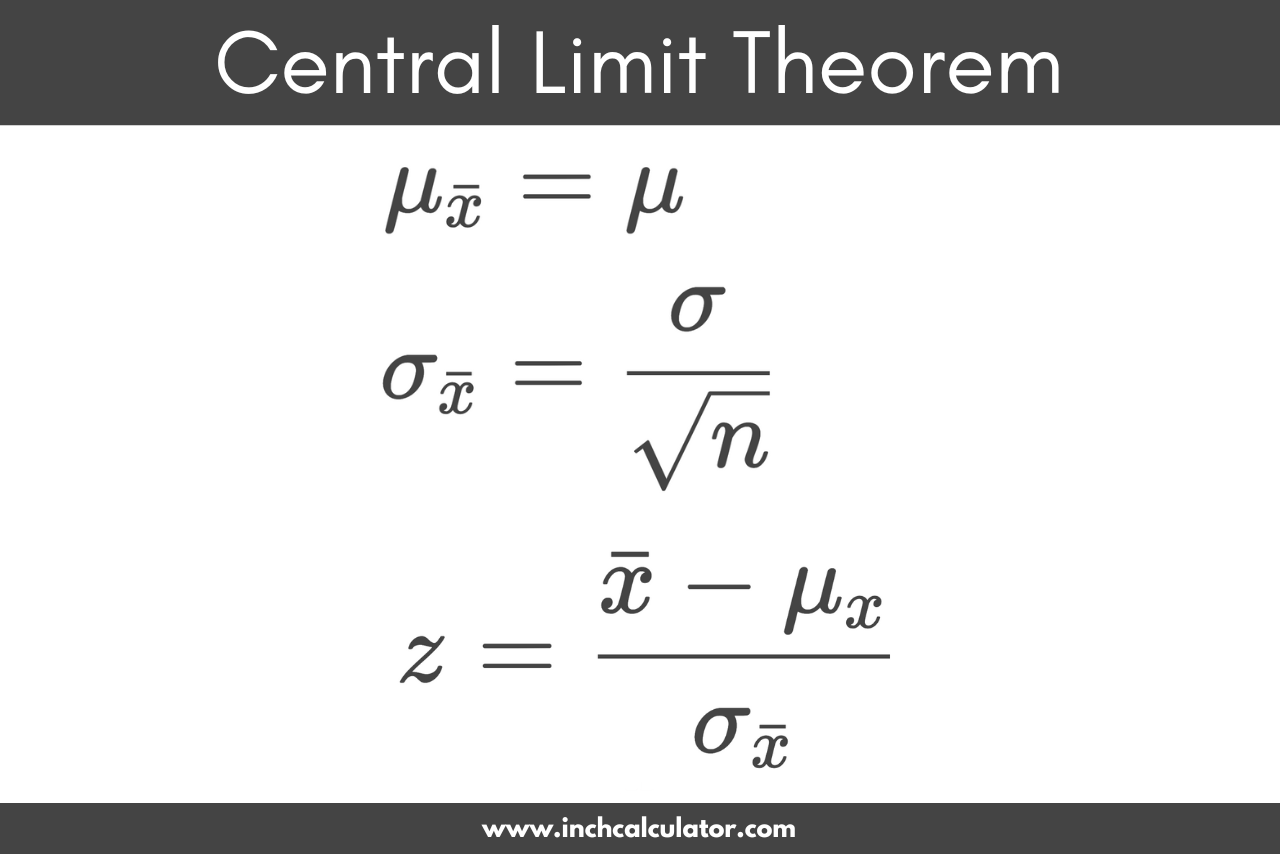# Central Limit Theorem Calculator

Enter the population mean, population standard deviation, and sample size to calculate the sample mean and standard deviation using the central limit theorem (CLT).

## Results:

### Steps to Solve Using the Central Limit Theorem

#### Find the Sample Mean

Provided the sample is sufficiently large, the sample mean will be equal to the population mean

μ = μ

#### Find the Sample Standard Deviation

The central limit theorem provides the following formula to solve the standard deviation for a sample

σ = σ / √n

Learn how we calculated this below

## How to Use the Central Limit Theorem

The central limit theorem for sample means states that as you take larger samples of independent random variables and calculate their means, the sample means form their own normal distribution, which is known as the sampling distribution of the mean. This distribution has the same mean as the original distribution and a variance equaling the original variance divided by the sample size.

Given a known population mean value and a sufficiently large sample, the central limit theorem dictates that the sample mean will equal the population mean. This holds true for sample sizes larger than 30.As you can see in the image above, as the sample size n increases, the values of each sample continue to be distributed around an equal mean.

### Central Limit Theorem Formula

Using the central limit theorem, you can calculate the mean, standard deviation, and z-score given a sufficiently large sample size with the following formulas.

Remember:
mean: The average of the data set.
standard deviation: The measure of how spread out the values of a dataset are from the mean.
z-score: The measure that quantifies how many standard deviations a specific data point is away from the mean of a distribution.Let’s dig into each of these in a little more detail.

#### Sample Mean Formula

You can use the central limit theorem to find the sample mean. If μ is the mean of the population values x, then μ is the mean of the sample values .

The sample mean μ is equal to the population mean μ.

μ = μ

For example, if the population mean μ is equal to 50, then a sample size of 100 will have a mean μ equal to 50 as well.

You can use our mean calculator to find the population mean μ.

#### Sample Standard Deviation Formula

You can also use the central limit theorem to find the standard deviation of a sample σ, given a known standard deviation of the population σ.

The central limit theorem provides the following formula to calculate the standard deviation of a sample.

σ = σ / √n

Thus, the central limit theorem states that the standard deviation of a sample σ is equal to the standard deviation of the population σ divided by the square root of the sample size n.

This is also referred to as the standard error of the mean.

For example, if the population standard deviation σ is equal to 2, let’s find the standard deviation σ of a sample with a size of 100.

σ = 2 / √100
σ = 2 / 10
σ = 0.2

So, this sample will have a standard deviation σ equal to 0.2.

You can use our standard deviation calculator to find the population standard deviation σ.

## How to Find Probabilities Using the Central Limit Theorem

The central limit theorem can also be used to find the probabilities of sample means. Start by using the following formula to find the z-score.

z = x̄ – μx / σ

The z-score z is equal to the sample mean minus μ, which is the average of x and , divided by the sample standard deviation σ.

Using the z-score, you can look up the probability of getting this mean using a z table.

By applying a margin of error and confidence interval, we can make estimates on an independent random variable given a data set with a sufficiently large sample size and assess how good those estimates are.

## References

1. McLeod, S., What is central limit theorem in statistics?, Simply Psychology, 11/25/2019, https://www.simplypsychology.org/central-limit-theorem.html
2. Illowsky, B., & Dean, S., The Central Limit Theorem for Sample Means (Averages), LibreTexts, 11/5/2021, https://stats.libretexts.org/@go/page/757